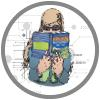## Cramming Sam's top tips from chapter 1

Click on the topic to read Sam's tips from the book

### Variables

When doing and reading research you’re likely to encounter these terms:

• Independent variable: A variable thought to be the cause of some effect. This term is usually used in experimental research to describe a variable that the experimenter has manipulated.
• Dependent variable: A variable thought to be affected by changes in an independent variable. You can think of this variable as an outcome.
• Predictor variable: A variable thought to predict an outcome variable. This term is basically another way of saying ‘independent variable’. (Although some people won’t like me saying that; I think life would be easier if we talked only about predictors and outcomes.)
• Outcome variable: A variable thought to change as a function of changes in a predictor variable. For the sake of an easy life this term could be synonymous with ‘dependent variable’.

### Levels of meaurement

• Variables can be split into categorical and continuous, and within these types there are different levels of measurement:
• Categorical (entities are divided into distinct categories):
• Binary variable: There are only two categories (e.g., dead or alive).
• Nominal variable: There are more than two categories (e.g., whether someone is an omnivore, vegetarian, vegan, or fruitarian).
• Ordinal variable: The same as a nominal variable but the categories have a logical order (e.g., whether people got a fail, a pass, a merit or a distinction in their exam).
• Continuous (entities get a distinct score):
• Interval variable: Equal intervals on the variable represent equal differences in the property being measured (e.g., the difference between 6 and 8 is equivalent to the difference between 13 and 15).
• Ratio variable: The same as an interval variable, but the ratios of scores on the scale must also make sense (e.g., a score of 16 on an anxiety scale means that the person is, in reality, twice as anxious as someone scoring 8). For this to be true, the scale must have a meaningful zero point.

### Central tendency

• The mean is the sum of all scores divided by the number of scores. The value of the mean can be influenced quite heavily by extreme scores.
• The median is the middle score when the scores are placed in ascending order. It is not as influenced by extreme scores as the mean.
• The mode is the score that occurs most frequently.

### Dispersion

• The deviance or error is the distance of each score from the mean.
• The sum of squared errors is the total amount of error in the mean. The errors/deviances are squared before adding them up.
• The variance is the average distance of scores from the mean. It is the sum of squares divided by the number of scores. It tells us about how widely dispersed scores are around the mean.
• The standard deviation is the square root of the variance. It is the variance converted back to the original units of measurement of the scores used to compute it. Large standard deviations relative to the mean suggest data are widely spread around the mean, whereas small standard deviations suggest data are closely packed around the mean.
• The range is the distance between the highest and lowest score.
• The interquartile range is the range of the middle 50% of the scores.

### Distributions and z-scores

• A frequency distribution can be either a table or a chart that shows each possible score on a scale of measurement along with the number of times that score occurred in the data.
• Scores are sometimes expressed in a standard form known as z-scores.
• To transform a score into a z-score you subtract from it the mean of all scores and divide the result by the standard deviation of all scores.
• The sign of the z-score tells us whether the original score was above or below the mean; the value of the z-score tells us how far the score was from the mean in standard deviation units.